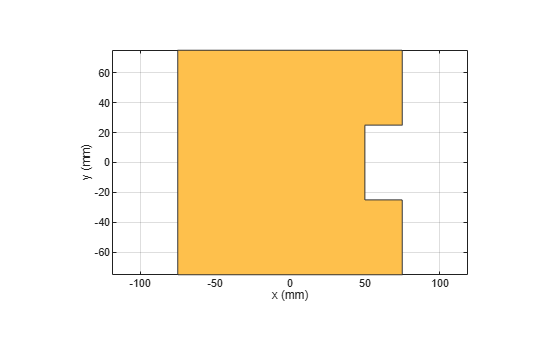# subtract

Boolean subtraction operation on two shapes

## Syntax

``c = subtract(shape1,shape2)``

## Description

example

````c = subtract(shape1,shape2)` subtracts `shape1` and `shape2` using the subtract operation. You can also use the `-` to subtract the two shapes.```

## Examples

collapse all

Create a rectangle with a length of 0.15 m, and a width of 0.15 m.

`r = antenna.Rectangle(Length=0.15,Width=0.15);`

Create a second rectangle with a length of 0.05 m, and a width of 0.05 m. Set the center of the second rectangle at half the length of the first rectangle r.

`n = antenna.Rectangle(Center=[0.075,0],Length=0.05,Width=0.05);`

Create and view a notched rectangle by subtracting n from r.

```rn = r-n; show(rn)```Calculate the area of the notched rectangle.

`area(rn)`
```ans = 0.0212 ```

## Input Arguments

collapse all

Shapes created using custom elements and shape objects of Antenna Toolbox™, specified as an object.

Example: `c = subtract(rectangle1, rectangle2)` where rectangle1 and rectangle2 are shapes created using `antenna.Rectangle` object.

## Version History

Introduced in R2017a